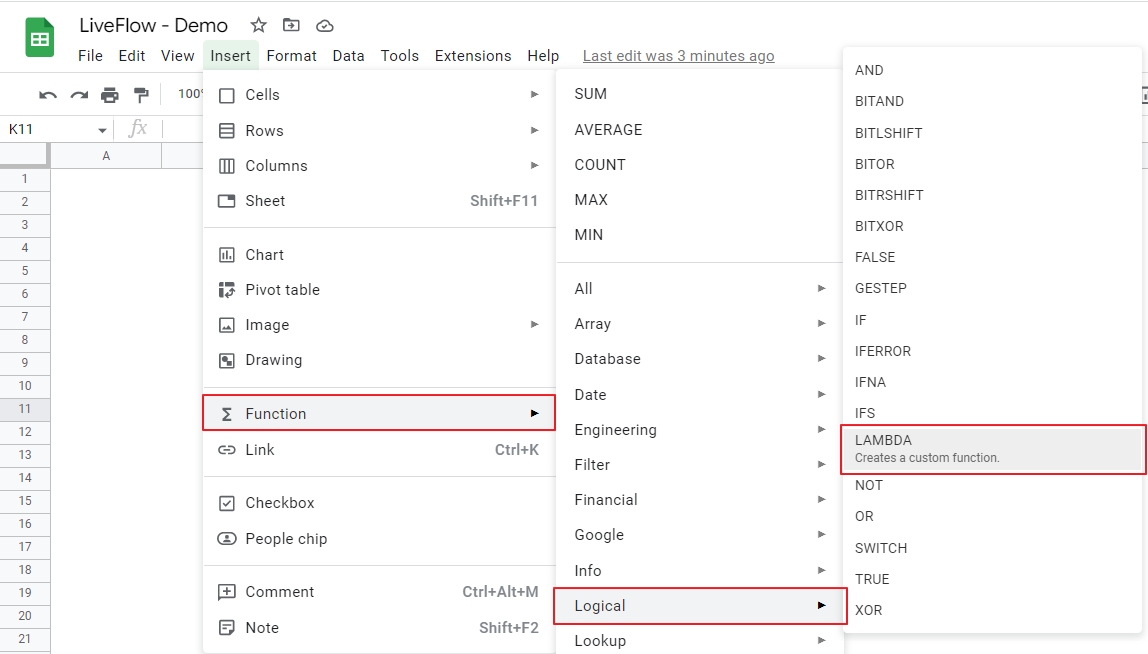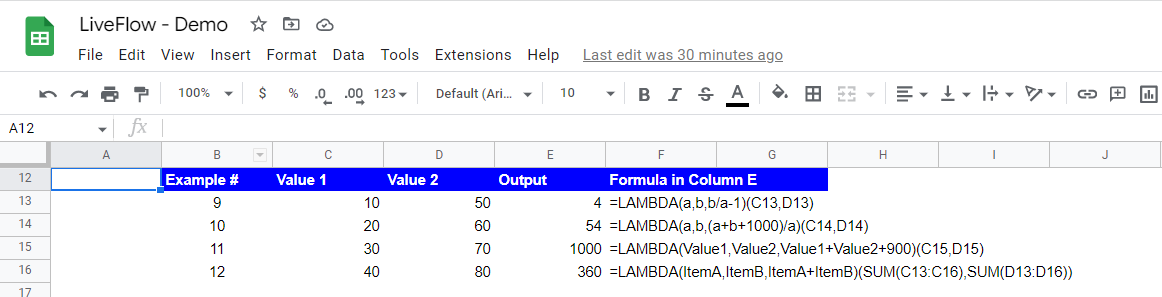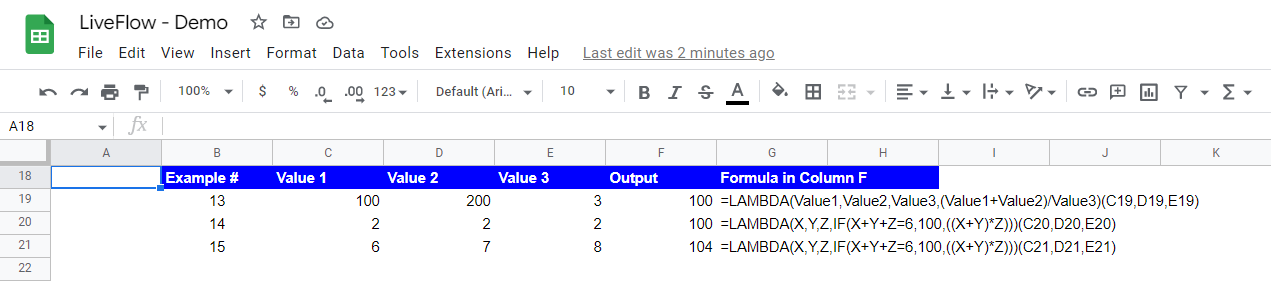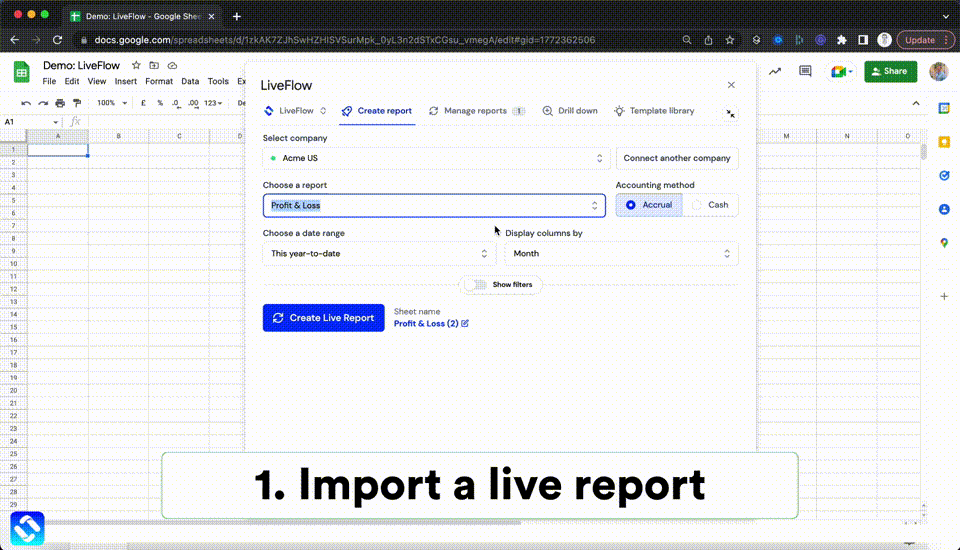# How to Use LAMBDA Function in Google Sheets

In this article, you will learn what the LAMBDA formula is and how to use it.

## What is the LAMBDA formula in Google Sheets?

The LAMBDA formula was introduced by Google in late August 2022. The function helps to create a customized formula with placeholders of values used in the formula. You can also share the customized formula with other collaborators by using the Named Function, which also became available in Google Sheets at the same time.

## How to use the LAMBDA formula in Google Sheets

1. Type “=LAMBDA” or go to “Insert”“Function” “Logical” → “LAMBDA”.
2. Determine, “name”, “formula_expression”, and “value”.
3. The formula returns the value computed by the formula you defined with the “value” input.How to insert the LAMBDA formula from the menu bar in Google Sheets

The generic formula of the LAMBDA is as follows:

``=LAMBDA([name, …], formula_expression)(value,...)``

Name: You define the names of the placeholders for values (including texts, numbers, and, dates, etc.)  that you incorporate in “formula_expression”. You can define them as much as you need by inserting commas between each of them.

Formula_expression: This is the part where you determine a formula including the placeholders defined in “Name” works. You need to define how each placeholder is processed, combined with general formulas such as “IF”.

Value: This is the actual value used when the formula defined in “formula_expression” runs its computation. The number of “values” should correspond to that of “name”. If you have more than one value, they can be punctuated by comma(s).

See the examples below. In these examples, the LAMBDA formula contains only one variable.

Example #1: In this example, “w” is a placeholder for an input value. As the value of cell C3 is 10, the formula returns 25 (=10+15=w+15).

Example #2: Similar to Example #1, “x” is a placeholder for an input value. As the value of cell C4 is 20, the formula returns 40 (=(20 multiplied 20) divided by 10=(x*x)/10). Another important point is that a placeholder can be referred repeatedly in the formula.

Examples #3 and #4: You can use other formulas when you determine the customized formula in the LAMBDA function. Here, we use the “IF” function. In this case, “y” is a placeholder for an actual value. The LAMBDA returns 30 when the input is cell C5 of 30 and gives you 90 when the input is cell C6 of 40, according to the IF formula contained.

Example #5: This example shows that a placeholder is not necessarily a single lowercase letter. You can use any word such as “Item” as a placeholder, too.

Examples #6 and #7: As you can see, you can include a text or a date in the “formula_expression” section. A placeholder need not be surrounded by quotations.

Examples #8: As shown in the screenshot, if you define “x” as a placeholder and if you use a different letter or word in the “formula_expression” part, the LAMBDA function won’t work. You need to refer to the defined placeholder name(s) in that part.

Next, see the examples below with two variables.How to use the LAMBDA with two variables in Google Sheets

Examples #9 to #11: These are the examples where you have two input values. Remember to punctuate two inputs with a comma.

Example #12: This is to show that you can incorporate a formula(s) as an input value. Apart from other examples shown, one of the values for this formula is SUM(C13:16)=100, and the other is SUM(D13:D16)=260. 100 corresponds to the placeholder of “ItemA” and 260 is tied to another placeholder of “ItemB”.

Finally, see the following examples with three placeholders.How to use the LAMBDA with three variables in Google Sheets

Examples #13 to #15: As the number of placeholders increases, the “formula_expression” looks more complicated, compared to the LAMBDA formulas with one or two variables. So, it is important to make the names of the placeholders as clear and simple as much as possible (e.g., giving them short and different names, etc.). Also, you should be careful about the correspondence of placeholders and values you input.

The examples you looked at in the screenshots are relatively simple and easy and you might think you don’t need to create a customized formula for them. However, as you saw various types of inputs in the LAMBDA formula here, you are ready to create a complicated customized formula when needed.

## Analyze your live financial data in a snap

Are you learning this formula to process financial data, build a financial model, or conduct financial analysis? If so, LiveFlow may help you to automate manual workflows and keep numbers updated in real time. For instance, you can easily compare your Budget versus Actual without any manual work. Furthermore, you can access more than fifty templates here, from the simple Income Statement and Operational KPI templates to Multi-Currency Consolidated Income Statement, Balance Sheet, and Cash Flow templates. You can also customize these templates as you want without breaking the automated data inflow.Automate any custom financial dashboard in Google Sheets with LiveFlow

Learn how to do this step-by-step in the video below 👇

## Do you need personal help?

Our team of real people are here to help you any time between 9am and 10pm GMT.Email us at: founders@liveflow.ioCall us at +1 (415) 650-1711